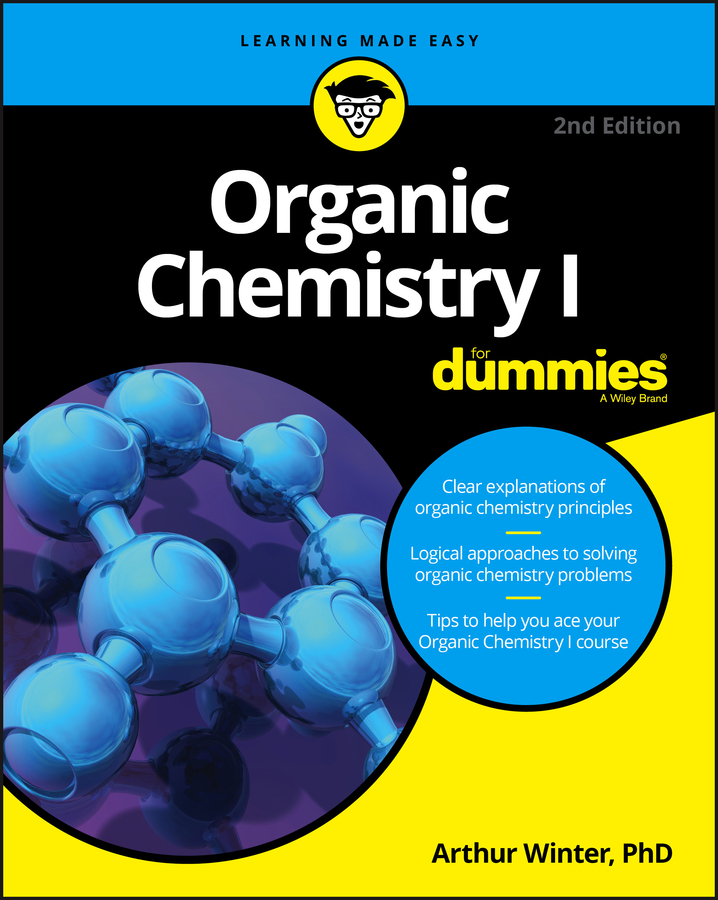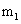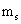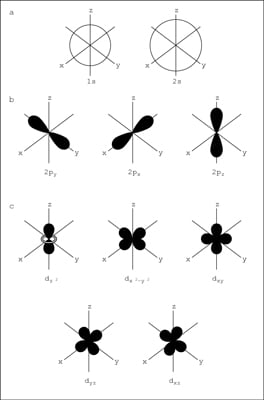##### Organic Chemistry I For DummiesTwo models of atomic structure are in use today: the Bohr model and the quantum mechanical model. The quantum mechanical model is based on mathematics. Although it is more difficult to understand than the Bohr model, it can be used to explain observations made on complex atoms.

A model is useful because it helps you understand what’s observed in nature. It’s not unusual to have more than one model represent and help people understand a particular topic.

The quantum mechanical model is based on quantum theory, which says matter also has properties associated with waves. According to quantum theory, it’s impossible to know the exact position and momentum of an electron at the same time. This is known as the Uncertainty Principle.

The quantum mechanical model of the atom uses complex shapes of orbitals (sometimes called electron clouds), volumes of space in which there is likely to be an electron. So, this model is based on probability rather than certainty.

Four numbers, called quantum numbers, were introduced to describe the characteristics of electrons and their orbitals:

• Principal quantum number: n

• Angular momentum quantum number: l

• Magnetic quantum number:• Spin quantum number:## The principal quantum number

The principal quantum number n describes the average distance of the orbital from the nucleus — and the energy of the electron in an atom. It can have positive integer (whole number) values: 1, 2, 3, 4, and so on. The larger the value of n, the higher the energy and the larger the orbital. Chemists sometimes call the orbitals electron shells.

## The angular momentum quantum number

The angular momentum quantum number l describes the shape of the orbital, and the shape is limited by the principal quantum number n: The angular momentum quantum number l can have positive integer values from 0 to n–1. For example, if the n value is 3, three values are allowed for l: 0, 1, and 2.

The value of l defines the shape of the orbital, and the value of n defines the size.

Orbitals that have the same value of n but different values of l are called subshells. These subshells are given different letters to help chemists distinguish them from each other. The following table shows the letters corresponding to the different values of l.

Letter Designations of the Subshells
Value of l (subshell) Letter
0 s
1 p
2 d
3 f
4 g

When chemists describe one particular subshell in an atom, they can use both the n value and the subshell letter — 2p, 3d, and so on. Normally, a subshell value of 4 is the largest needed to describe a particular subshell. If chemists ever need a larger value, they can create subshell numbers and letters.

The following figure shows the shapes of the s, p, and d orbitals.As shown in the top row of the figure (a), there are two s orbitals — one for energy level 1 (1s) and the other for energy level 2 (2s). The s orbitals are spherical with the nucleus at the center. Notice that the 2s orbital is larger in diameter than the 1s orbital. In large atoms, the 1s orbital is nestled inside the 2s, just like the 2p is nestled inside the 3p.

The second row of the figure (b) shows the shapes of the p orbitals, and the last two rows (c) show the shapes of the d orbitals. Notice that the shapes get progressively more complex.

## The magnetic quantum number

The magnetic quantum number is designated as:This number describes how the various orbitals are oriented in space. The value of this number depends on the value of l. The values allowed are integers from –l to 0 to +l. For example, if the value of l = 1 (p orbital), you can write three values for this number: –1, 0, and +1. This means that there are three different p subshells for a particular orbital. The subshells have the same energy but different orientations in space.

The second row (b) of the figure shows how the p orbitals are oriented in space. Notice that the three p orbitals correspond to magnetic quantum number values of –1, 0, and +1, oriented along the x, y, and z axes.

## The spin quantum number

The fourth and final quantum number is the spin quantum number, designated as:This number describes the direction the electron is spinning in a magnetic field — either clockwise or counterclockwise. Only two values are allowed: +1/2 or –1/2. For each subshell, there can be only two electrons, one with a spin of +1/2 and another with a spin of –1/2.